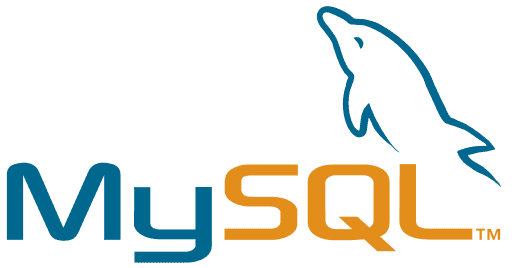since 1999

# Best Data Type to store Money in MySQL?### If GAAP Compliance is required or you need 4 decimal places:

``````DECIMAL(13, 4)
``````

Which supports a max value of:

`\$999,999,999.9999`

### Otherwise, if 2 decimal places is enough:

``````DECIMAL(13,2)
``````

Which supports a max value of:

`\$99,999,999,999.99`

## Too Short; Want More

Some relational databases support a money type, but we aren’t as lucky when it comes to MySQL. This leaves the choice of data type to the developer.

Since MySQL 5 we have the luxury of using `DECIMAL` as a datatype.

Note that `DECIMAL` and `NUMERIC` are the same datatype.

From the MySQL manual:

The DECIMAL and NUMERIC types store exact numeric data values. These types are used when it is important to preserve exact precision, for example with monetary data. In MySQL, NUMERIC is implemented as DECIMAL, so the following remarks about DECIMAL apply equally to NUMERIC.

The `DECIMAL` data type allows you to specify the total number of digits as well as the number of digits after the decimal point. If no values are specified, the default is `DECIMAL(10,0)` which is not suitable for money.

If you need to support more than the max value stated above, you can increase the first argument (13 in the example) to fit your needs. Likewise, the second number of `DECIMAL` increases the number of digits after the decimal point.

If the application needs to handle money values up to a trillion dollars (or any other currency), then use 2 decimal places:

``````  DECIMAL(13, 2)
``````

If you need to comply with GAAP, then use 4 decimal places:

``````  DECIMAL(13, 4)
``````

When displaying this value to the end user, finish all mathematical operations utilizing the more accurate 4 decimal places, then round the final result to two decimal places (`ROUND(number, 2)` in MySQL). GAAP is concerned with ensuring numerical rounding errors are small enough to be considered non-material even when dealing with many entries.